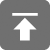# 工学1号馆

home

## HDOJ 1003 Max Sum

Wu Yudong    August 17, 2018     HDOJ   543

Problem Description
Given a sequence a,a,a......a[n], your job is to calculate the max sum of
a sub-sequence. For example, given (6,-1,5,4,-7), the max sum in this sequence
is 6 + (-1) + 5 + 4 = 14.

Input
The first line of the input contains an integer T(1<=T<=20) which means the number of
test cases. Then T lines follow, each line starts with a number N(1<=N<=100000),
then N integers followed(all the integers are between -1000 and 1000).

Output
For each test case, you should output two lines.
The first line is "Case #:", # means the number of the test case.
The second line contains three integers, the Max Sum in the sequence,
the start position of the sub-sequence, the end position of the sub-sequence.
If there are more than one result, output the first one. Output a blank line between
two cases.

Sample Input
2
5 6 -1 5 4 -7
7 0 6 -1 1 -6 7 -5

Sample Output
Case 1:
14 1 4

Case 2:
7 1 6


dp[ i + 1] = max(dp[ i ] + a[ i + 1], a[ i + 1])

#include<stdio.h>

int main()
{
int t, n, num, sum, max, begin, end, temp, count, i;
scanf("%d", &t); //输入例子数
for (count = 1; count <= t; count++) {
scanf("%d", &n); //输入数组长度
sum = 0;
temp = 1; //存储新的起始位置
end = 1;
max = -1001; //存储最大值
for (i = 1; i <= n; i++) {
scanf("%d", &num);
sum += num;
if (sum < num) {
temp = i;
sum = num;
}
if (sum > max) {
max = sum;
begin = temp;
end = i;
}

}
printf("Case %d:\n%d %d %d\n", count, max, begin, end);
if (count != t)
printf("\n");
}
return 0;

}